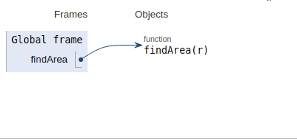# Python Program to find the area of a circle

In this article, we will learn about the solution and approach to solve the given problem statement.

Problem statement −Given the radius of a circle, we need to find a circle.

The area of a circle can simply be evaluated using the following formula.

Area = Pi*r*r

Let’s see the implementation below −

## Example

Live Demo

def findArea(r):
PI = 3.142
return PI * (r*r);
# Driver method
print("Area is %.6f" % findArea(5));

## Output

Area is 78.550000

All variables and functions are declared in the global scope as shown in the figure below.## Conclusion

In this article, we learned about the approach to find whether it is possible to make a divisible by 3 numbers using all digits in an array.